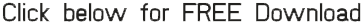TutorialsPublished by : 0nelove | Date : 11-07-2019 | Views : 122
Python Application Series: Machine Learning Using TensorflowPython Application Series: Machine Learning Using Tensorflow
MP4 | Video: AVC 1018x572 | Audio: AAC 44kHz 2ch | Duration: 3.5 Hours | 778 MB
Genre: eLearning | Language: English

The following 10 topics will be covered through a combination of lecture and hands-on to maximize your mastery of machine learning using Tensorflow:

Introducing Tensorflow. This first topic in the Machine Learning using Tensorflow series introduces you to the world of Tensorflow. Learn about the history of Tensorflow, Tensorflow's architecture, and the various components of Tensorflow. Appreciate the reasons why Tensorflow is so popular. Follow along with Rohit as he shows you how to download Tensorflow and where to find the official Tensorflow documentation.
Setting up the Tensorflow Environment. This second video in the Machine Learning using Tensorflow series explains how to install and set up the Tensorflow environment on the Linux, Windows (using Anaconda), and Mac operating systems. Follow along with Rohit as he demonstrates the power of Tensorflow, and learn about the Tensorflow-GPU.
Applying Artificial Intelligence, Machine Learning, and Deep Learning. This third video in the Machine Learning using Tensorflow series explains artificial intelligence (AI), machine learning, and deep learning (both supervised and unsupervised).
Applying Tensorflow Basics. This fourth video in the Machine Learning using Tensorflow series explains how to apply the basic mathematical scalar, vector, and matrix functionality in Tensorflow. Learn all about the concepts of tensor, rank, shape, type, graph, session, variable, and placeholder. Follow along with Rohit in this hands-on session using Python.
Mastering Numpy with Tensorflow. This fifth video in the Machine Learning using Tensorflow series covers the Python package Numpy, and how it can be used with Tensorflow. Practice working with Numpy attributes (including shape, reshape, arrange, and item size) and Numpy arrays (including empty, zeros, and ones). Follow along with Rohit in this hands-on session using Python.
Mastering Pandas with Tensorflow. This sixth video in the Machine Learning using Tensorflow series explains how to work with Pandas, and how Pandas can be used with Tensorflow. Follow along with Rohit in this hands-on session using Python.
Mastering Matplotlib with Tensorflow. This seventh video in the Machine Learning using Tensorflow series explains how to create Matplotlib graphs, and how Matplotlib graphs can be used with Tensorflow. Follow along with Rohit in this hands-on session using Python.
Implementing Neural Networks using Tensorflow. This eighth video in the Machine Learning using Tensorflow series explains how to implement neural networks using Tensorflow. Follow along with Rohit in this hands-on session using Python.
Implementing Convolutional Neural Networks using Tensorflow. This ninth video in the Machine Learning using Tensorflow series explains how to implement Convolutional Neural Networks (CNNs) using Tensorflow. Learn all about convolution, non linearity (ReLU), pooling, sub sampling, and classification. Follow along with Rohit in this hands-on session using Python.
Implementing Recurrent Neural Networks using Tensorflow. This tenth video in the Machine Learning using Tensorflow series explains how to implement Recurrent Neural Networks (RNNs) using Tensorflow. Follow along with Rohit in this hands-on session using Python.

Enjoy!

http://nitroflare.com/view/EC8E4C7948E84E0/Python_Application_Series_-_Machine_Learning_using_Tensorflow.rar

or
https://rapidgator.net/file/51c3c05865e946243968fcd222695902/Python_Application_Series_-_Machine_Learning_using_Tensorflow.rar.html